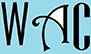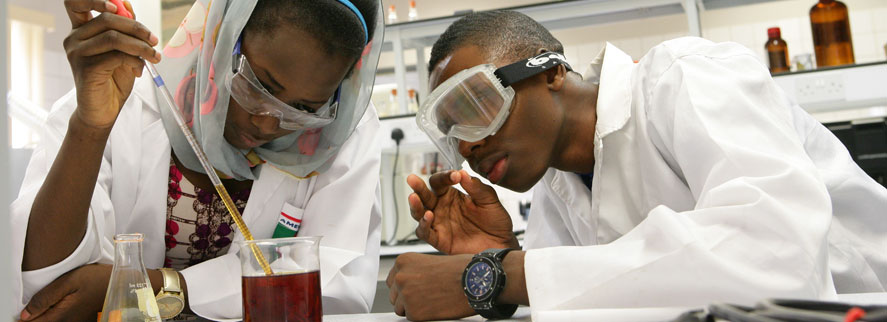## Algebra Question for Title 1 Schools: Percent of that Number Question?

18) If 45 is 120% of a number, what is 80% of that number?

120/100 x y = 45

of = x (multiply)

you have to make “y” subject of formula by moving “100” and “120” to the other side. When you go to the other side, addition becomes subtraction, multiplication becomes division, and vice versa.

y = 45 x 100/120

y = 4500/120

y = 225/6

y = 37.5

80% = 0.8

37.5 x 0.8  = 30